Courses

# Test: Arithmetic Mean Of Grouped Data

## 15 Questions MCQ Test Mathematics (Maths) Class 10 | Test: Arithmetic Mean Of Grouped Data

Description
This mock test of Test: Arithmetic Mean Of Grouped Data for Class 10 helps you for every Class 10 entrance exam. This contains 15 Multiple Choice Questions for Class 10 Test: Arithmetic Mean Of Grouped Data (mcq) to study with solutions a complete question bank. The solved questions answers in this Test: Arithmetic Mean Of Grouped Data quiz give you a good mix of easy questions and tough questions. Class 10 students definitely take this Test: Arithmetic Mean Of Grouped Data exercise for a better result in the exam. You can find other Test: Arithmetic Mean Of Grouped Data extra questions, long questions & short questions for Class 10 on EduRev as well by searching above.
QUESTION: 1

### The mean of five numbers is 28. If one of the numbers is excluded, the mean gets reduced by 2. The excluded number is

Solution:

Mean of 5 nos.= 28

Therefore, sum of the 5 nos. = 28 * 5 = 140

The the no. exclude by 'x'.

New sum of 4 nos.= 140 - x

New mean = 28- 2 = 26

Therefore, (140 - x ) / 4 = 26

=> 140 - x = 104

=> x = 36

QUESTION: 2

Solution:
QUESTION: 3

### The mean of the following data is: 45, 35, 20, 15, 25, 40​

Solution:
QUESTION: 4

The mean of 5 observations x, x + 2, x + 4, x + 6 and x + 8 is 11, then the value of x is:

Solution:

(x + x + 2 + x + 4 + x + 6 + x + 8) / 5 = 11

5x + 20 =  55

5x = 35 ⇒ x = 7

QUESTION: 5

The mean of a data set with 12 observations is calculated as 19.25. If one more value is included in the data, then for the new data with 13 observations, mean becomes 20. Value of this 13th observation is​

Solution:

Suppose be all twelevth terms = x
Mean = Terms / Observation
19 . 25 = x / 12
x = 19.25 × 12
x = 231 .00

Now let thirteenth term will be y
Mean = 20
So, 20 = x + y / 13
20 = 231 + y / 13
20 × 13 = 231 + x
260 = 231 + x
260 - 231 = x
29 = x

QUESTION: 6

The mean of first ten even natural numbers is​

Solution:
QUESTION: 7

A batsman in his 12th innings makes a score of 63 runs and thereby increases his average score by 2. His average score after 12th innings is​

Solution:
QUESTION: 8

Which measure of central tendency takes in account all the data?​

Solution:
QUESTION: 9

The mean (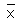) of a frequency distribution is 45. If the value of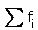= 20 then the value of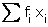is:

Solution:
QUESTION: 10

The mean of a data set with 12 observations is calculated as 19.25. If one more value is included in the data, then for the new data with 13 observations, mean becomes 20. The value of this 13th observation is​

Solution:
QUESTION: 11

In the following distribution, if the mean of the distribution is 86 then the value of p is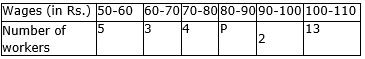Solution:
QUESTION: 12

The mean of 1, 2, 3, 4 …n is given by

Solution:

⇒   We have the sequence, 1,2,3.......n
⇒   This is an AP, with the initial term a=1 and the common difference d=1.
∴   The sum of n terms of an AP is given by,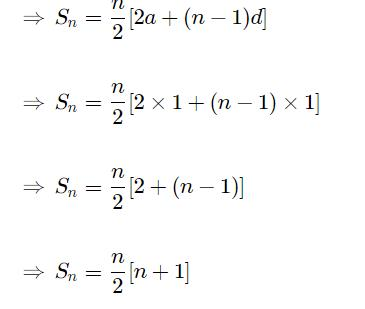→   Arithmetic mean of n numbers a1​,a2​,a3​,a4​,...an​ is given by the formula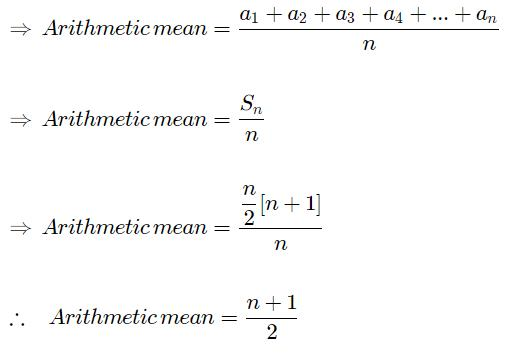QUESTION: 13

The arithmetic mean of the following data is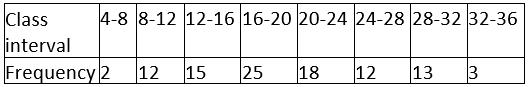Solution:
QUESTION: 14

The mean of the marks obtained by 7 students in a group is 226. If the marks obtained by six of them are 340, 180, 260, 56, 275, 307, then the marks obtained by the seventh student are​

Solution:
QUESTION: 15

The mean of 15 numbers is 25. If each number is multiplied by 4, mean of the new numbers is

Solution: